Open in App
Not now

# How to randomly select rows from Pandas DataFrame

• Difficulty Level : Easy
• Last Updated : 23 Jan, 2022

Let’s discuss how to randomly select rows from Pandas DataFrame. A random selection of rows from a DataFrame can be achieved in different ways.
Create a simple dataframe with dictionary of lists.

## Python3

 `# Import pandas package` `import` `pandas as pd` ` `  `# Define a dictionary containing employee data` `data ``=` `{``'Name'``:[``'Jai'``, ``'Princi'``, ``'Gaurav'``, ``'Anuj'``, ``'Geeku'``],` `        ``'Age'``:[``27``, ``24``, ``22``, ``32``, ``15``],` `        ``'Address'``:[``'Delhi'``, ``'Kanpur'``, ``'Allahabad'``, ``'Kannauj'``, ``'Noida'``],` `        ``'Qualification'``:[``'Msc'``, ``'MA'``, ``'MCA'``, ``'Phd'``, ``'10th'``]}`   `# Convert the dictionary into DataFrame ` `df ``=` `pd.DataFrame(data)`   `# select all columns` `df`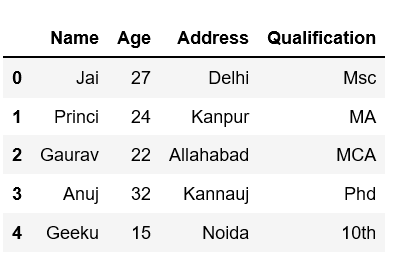Method #1: Using sample() method

Sample method returns a random sample of items from an axis of object and this object of same type as your caller.

Example 1:

## Python3

 `# Selects one row randomly using sample() ` `# without give any parameters.`   `# Import pandas package` `import` `pandas as pd` ` `  `# Define a dictionary containing employee data` `data ``=` `{``'Name'``:[``'Jai'``, ``'Princi'``, ``'Gaurav'``, ``'Anuj'``, ``'Geeku'``],` `        ``'Age'``:[``27``, ``24``, ``22``, ``32``, ``15``],` `        ``'Address'``:[``'Delhi'``, ``'Kanpur'``, ``'Allahabad'``, ``'Kannauj'``, ``'Noida'``],` `        ``'Qualification'``:[``'Msc'``, ``'MA'``, ``'MCA'``, ``'Phd'``, ``'10th'``]}`   `# Convert the dictionary into DataFrame ` `df ``=` `pd.DataFrame(data)`   `# Select one row randomly using sample()` `# without give any parameters` `df.sample()`

Output: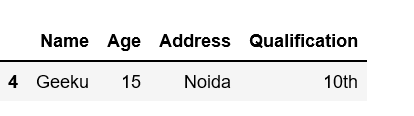Example 2: Using parameter n, which selects n numbers of rows randomly.
Select n numbers of rows randomly using sample(n) or sample(n=n). Each time you run this, you get n different rows.

## Python3

 `# To get 3 random rows` `# each time it gives 3 different rows`   `# df.sample(3) or` `df.sample(n ``=` `3``)`

Output: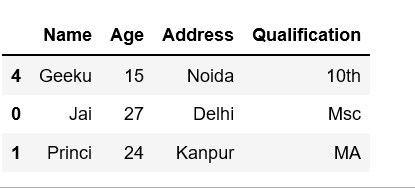Example 3: Using frac parameter.
One can do fraction of axis items and get rows. For example, if frac= .5 then sample method return 50% of rows.

## Python3

 `# Fraction of rows`   `# here you get .50 % of the rows` `df.sample(frac ``=` `0.5``)`

Output: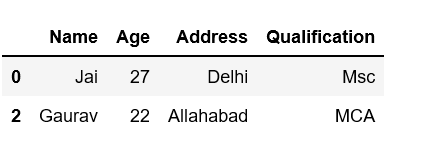Example 4:
First selects 70% rows of whole df dataframe and put in another dataframe df1 after that we select 50% frac from df1

## Python3

 `# fraction of rows`   `# here you get 70 % row from the df` `# make put into another dataframe df1` `df1 ``=` `df.sample(frac ``=``.``7``)`   `# Now select 50 % rows from df1` `df1.sample(frac ``=``.``50``)`

Output: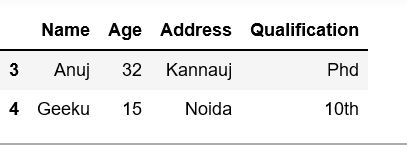Example 5: Select some rows randomly with replace = false
Parameter replace give permission to select one rows many time(like). Default value of replace parameter of sample() method is False so you never select more than total number of rows.

## Python3

 `# Dataframe df has only 4 rows`   `# if we try to select more than 4 row then will come error ` `# Cannot take a larger sample than population when 'replace = False'` `df1.sample(n ``=` `3``, replace ``=` `False``)`

Output: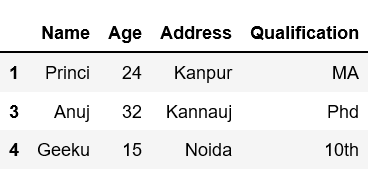Example 6: Select more than n rows where n is total number of rows with the help of replace.

## Python3

 `# Select more than rows with using replace` `# default it is False ` `df1.sample(n ``=` `6``, replace ``=` `True``)`

Output: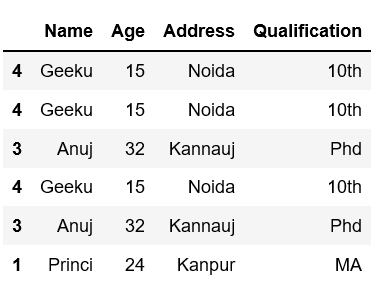Example 7: Using weights

## Python3

 `# Weights will be re-normalized automatically` `test_weights ``=` `[``0.2``, ``0.2``, ``0.2``, ``0.4``]`   `df1.sample(n ``=` `3``, weights ``=` `test_weights)`

Output: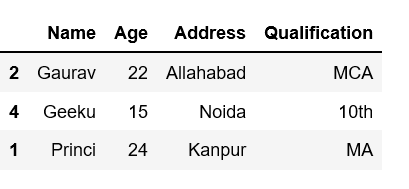Example 8: Using axis
The axis accepts number or name. sample() method also allows users to sample columns instead of rows using the axis argument.

## Python3

 `# Accepts axis number or name. `   `# sample also allows users to sample columns` `# instead of rows using the axis argument.` `df1.sample(axis ``=` `0``)`

Output: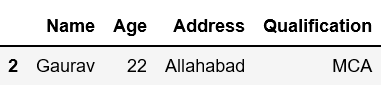Example 9: Using random_state
With a given DataFrame, the sample will always fetch same rows. If random_state is None or np.random, then a randomly-initialized RandomState object is returned.

## Python3

 `# With a given seed, the sample will always draw the same rows.`   `# If random_state is None or np.random,` `# then a randomly-initialized` `# RandomState object is returned.` `df1.sample(n ``=` `2``, random_state ``=` `2``)`

Output: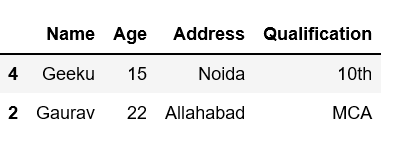Method #2: Using NumPy
Numpy choose how many index include for random selection and we can allow replacement.

## Python3

 `# Import pandas & Numpy package` `import` `numpy as np` `import` `pandas as pd` ` `  `# Define a dictionary containing employee data` `data ``=` `{``'Name'``:[``'Jai'``, ``'Princi'``, ``'Gaurav'``, ``'Anuj'``, ``'Geeku'``],` `        ``'Age'``:[``27``, ``24``, ``22``, ``32``, ``15``],` `        ``'Address'``:[``'Delhi'``, ``'Kanpur'``, ``'Allahabad'``, ``'Kannauj'``, ``'Noida'``],` `        ``'Qualification'``:[``'Msc'``, ``'MA'``, ``'MCA'``, ``'Phd'``, ``'10th'``]}`   `# Convert the dictionary into DataFrame ` `df ``=` `pd.DataFrame(data)`   `# Choose how many index include for random selection` `chosen_idx ``=` `np.random.choice(``4``, replace ``=` `True``, size ``=` `6``)`   `df2 ``=` `df.iloc[chosen_idx]`   `df2`

Output: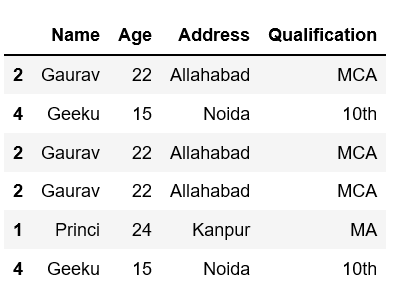My Personal Notes arrow_drop_up
Related Articles